> 文档中心 > 机器学习实战运用：速刷牛客5道机器学习题目

# 机器学习实战运用：速刷牛客5道机器学习题目

解答

四、AI4 决策树的生成与训练-信息增益

解答：

五、AI5 使用梯度下降对逻辑回归进行训练

Logistic模型原理详解以及Python项目实现

# 前言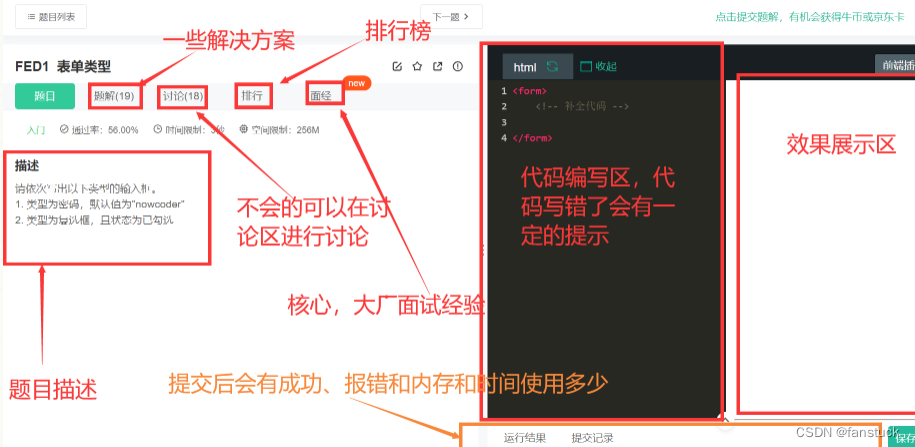# 一、AI1 鸢尾花分类_1

## 描述：

train_and_predict函数接收三个参数:

• train_input_features—二维NumPy数组，其中每个元素都是一个数组，它包含:萼片长度、萼片宽度、花瓣长度和花瓣宽度。

• train_outputs—一维NumPy数组，其中每个元素都是一个数字，表示在train_input_features的同一行中描述的鸢尾花种类。0表示鸢尾setosa，1表示versicolor，2代表Iris virginica。

• prediction_features—二维NumPy数组，其中每个元素都是一个数组，包含:萼片长度、萼片宽度、花瓣长度和花瓣宽度。

## 解答：

``import numpy as npfrom sklearn import datasetsfrom sklearn.model_selection import train_test_splitfrom sklearn import metricsfrom sklearn.ensemble import RandomForestClassifier def train_and_predict(train_input_features, train_outputs, prediction_features):  #code start here    rfc = RandomForestClassifier(random_state=0)  #随机森林    rfc = rfc.fit(train_input_features,train_outputs)#训练    prediction_result=rfc.predict(prediction_features)    return prediction_result    #code end hereiris = datasets.load_iris()X_train, X_test, y_train, y_test = train_test_split(iris.data, iris.target,\   test_size=0.3, random_state=0)y_pred = train_and_predict(X_train, y_train, X_test)if y_pred is not None: #code start here    print(metrics.accuracy_score(y_test, y_pred))    #code end here``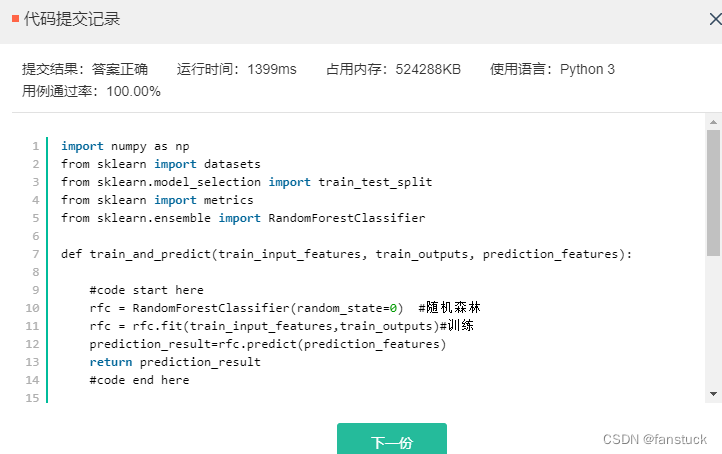# 二、AI2 鸢尾花分类_2

## 解答

``import numpy as npfrom sklearn import datasetsfrom sklearn.model_selection import train_test_splitfrom sklearn.preprocessing import MinMaxScalerfrom sklearn.linear_model import LogisticRegressionfrom sklearn.metrics import f1_score,roc_auc_score,accuracy_scorefrom sklearn.tree import DecisionTreeClassifierdef transform_three2two_cate():    data = datasets.load_iris()    #其中data特征数据的key为data，标签数据的key为target    #需要取出原来的特征数据和标签数据，移除标签为2的label和特征数据，返回值new_feat为numpy.ndarray格式特征数据，    #new_label为对应的numpy.ndarray格式label数据    #需要注意特征和标签的顺序一致性，否则数据集将混乱    #code start here    index_2=np.where(np.array(data.target==2))    new_feat=np.delete(data.data,index_2,0)    new_label=np.delete(data.target,index_2)    #code end here    return new_feat,new_labeldef train_and_evaluate():    data_X,data_Y = transform_three2two_cate()    train_x,test_x,train_y,test_y = train_test_split(data_X,data_Y,test_size = 0.2)    #已经划分好训练集和测试集，接下来请实现对数据的训练    #code start here    classifier = LogisticRegression(solver='liblinear',C=100)    classifier.fit(train_x, train_y)    y_predict=classifier.predict(test_x)    #code end here    #注意模型预测的label需要定义为 y_predict，格式为list或numpy.ndarray    print(accuracy_score(y_predict,test_y))if __name__ == "__main__":    train_and_evaluate()    #要求执行train_and_evaluate()后输出为：    #1、{0,1}，代表数据label为0和1    #2、测试集上的准确率分数，要求>0.95``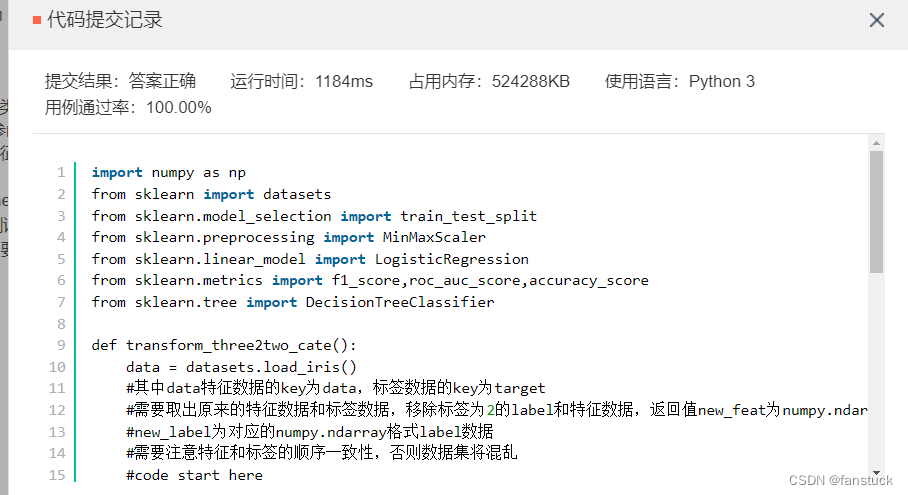# 三、 AI3 决策树的生成与训练-信息熵的计算

## 描述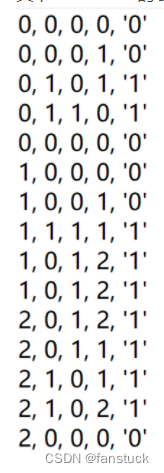## 解答

``# -*- coding: UTF-8 -*-from math import logimport pandas as pddataSet = pd.read_csv('dataSet.csv', header=None).values.tolist()def calcInfoEnt(dataSet):    numEntres = len(dataSet)    #code start here    labelCounts = {} #创建记录不同分类标签结果多少的字典    #为所有可能分类保存    #该字典key：label value:label的数目    for featVec in dataSet: currentLabel = featVec[-1] if currentLabel not in labelCounts.keys():     labelCounts[currentLabel] = 0 labelCounts[currentLabel] += 1    infoEnt = 0.0    for key in labelCounts: prob = float(labelCounts[key])/numEntres #标签发生概率p(xi)的值 infoEnt -= prob * log(prob,2)    return infoEnt    #code end here    #返回值 infoEnt 为数据集的信息熵，表示为 float 类型    if __name__ == '__main__':    print(calcInfoEnt(dataSet))    #输出为当前数据集的信息熵``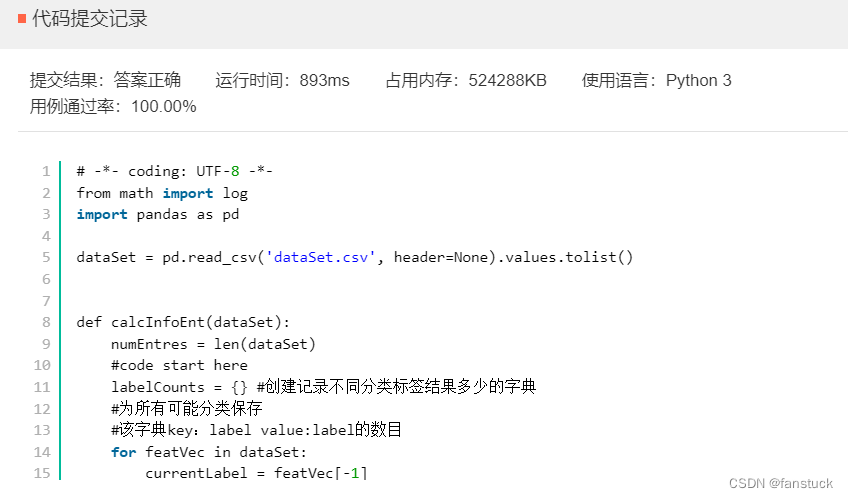# 四、AI4 决策树的生成与训练-信息增益

## 描述

第二步是计算每个特征的信息增益，特征 A 对于数据集 D 的经验条件熵可以表示为：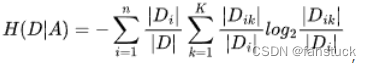其中CK代表的是属于某一类的样本个数，D 是整个数据集的样本数量，根据某一特征不同取值可以将数据划分为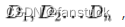其中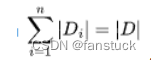K 为类别的数目，某一特征的信息增益即为信息熵和经验条件熵的差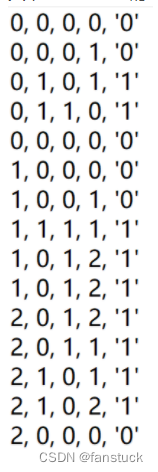## 选择最优划分算法

``# -*- coding: UTF-8 -*-from math import logimport pandas as pddataSet = pd.read_csv('dataSet.csv', header=None).values.tolist()#给定一个数据集，calcInfoEnt可以用于计算一个数据集的信息熵，可直接调用#也可不使用，通过自己的方式计算信息增益def calcInfoEnt(data):    numEntres = len(data)    labelcnt = {} #用于统计正负样本的个数    for item in data: if item[-1] not in labelcnt:     labelcnt[item[-1]] = 0 labelcnt[item[-1]] += 1    infoEnt = 0.0    for item in labelcnt: #根据信息熵的公式计算信息熵 curr_info_entr = float(labelcnt[item]) / numEntres infoEnt = infoEnt - curr_info_entr * log(curr_info_entr,2)    return infoEnt    #返回值 infoEnt 为数据集的信息熵#给定一个数据集，用于切分一个子集，可直接用于计算某一特征的信息增益 #也可不使用，通过自己的方式计算信息增益   #dataSet是要划分的数据集，i 代表第i个特征的索引index#value对应该特征的某一取值def create_sub_dataset(dataSet, i, value):     res = []    for item in dataSet: if item[i] == value:     curr_data = item[:i] + item[i+1:]     res.append(curr_data)    return resdef calc_max_info_gain(dataSet):#计算所有特征的最大信息增益，dataSet为给定的数据集    n = len(dataSet)-1  # n 是特征的数量，-1 的原因是最后一列是分类标签    total_entropy = calcInfoEnt(dataSet)#整体数据集的信息熵    max_info_gain =[0,0.0]#返回值初始化    #code start here    bestFeature = -1    for i in range(n): #创建唯一的分类标签列表 featList = [example[i] for example in dataSet] uniqueVals = set(featList) #建立列表同特征下不同回答 newEntropy = 0.0 #计算每种划分方式的信息熵 for value in uniqueVals:     subDataSet = create_sub_dataset(dataSet,i,value) #划分     prob = len(subDataSet)/float(len(dataSet)) #同特征下不同回答所占总回答比率     newEntropy += prob * calcInfoEnt(subDataSet) #该特征划分下的信息熵 infoGain = total_entropy - newEntropy #信息增益 if ( infoGain > max_info_gain ):  max_info_gain =infoGain  max_info_gain=i  bestFeature = i    #code end here    return max_info_gainif __name__ == '__main__':    info_res = calc_max_info_gain(dataSet)    print("信息增益最大的特征索引为：{0},对应的信息增益为{1}".format(info_res,info_res))``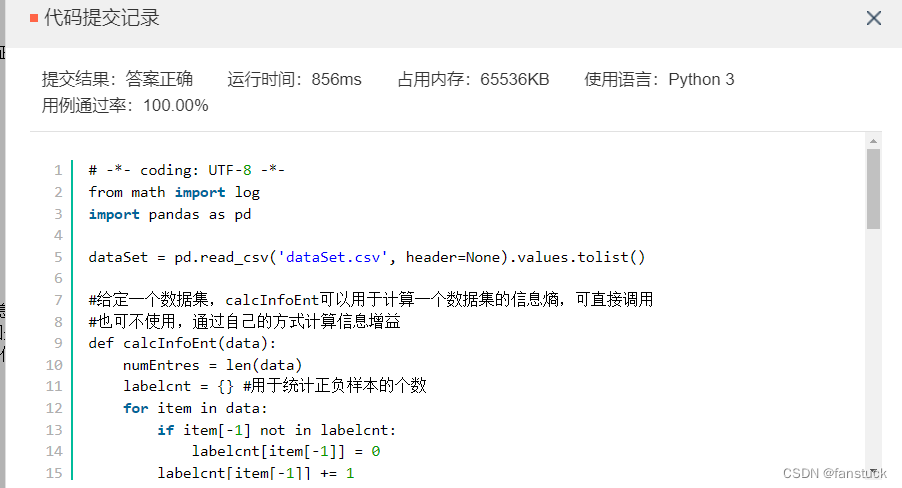# 五、AI5 使用梯度下降对逻辑回归进行训练

## 描述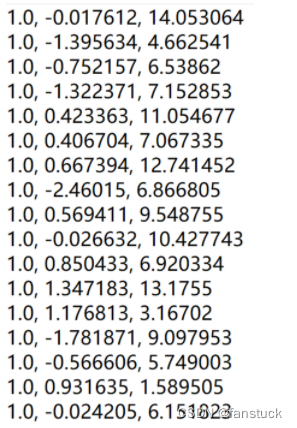lables.csv示例数据集如下：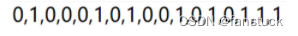## Logistic模型原理详解以及Python项目实现

• 1）步长（learning rate）：步长决定了在梯度下降迭代过程中，每一步沿梯度负方向前进的长度
• 2）特征（feature）：指的是样本中输入部门，比如样本（x0，y0），（x1，y1），则样本特征为x，样本输出为y
• 3）假设函数（hypothesis function）：在监督学习中，为了拟合输入样本，而使用的假设函数，记为。比如对于样本（,）(i=1,2,...n),可以采用拟合函数如下：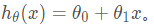• 4）损失函数（loss function）：为了评估模型拟合的好坏，通常用损失函数来度量拟合的程度。损失函数极小化，意味着拟合程度最好，对应的模型参数即为最优参数。在线性回归中，损失函数通常为样本输出和假设函数的差取平方。比如对于样本（,）(i=1,2,...n),采用线性回归，损失函数为：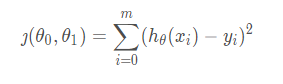其中​表示样本特征x的第i个元素，表示样本输出y的第i个元素， 为假设函数。

。该公式停止的条件是迭代次数达到某个指定值或者算法达到某个允许的误差范围。

``import numpy as npimport pandas as pddef generate_data():    datasets = pd.read_csv('dataSet.csv', header=None).values.tolist()    labels = pd.read_csv('labels.csv', header=None).values.tolist()    return datasets, labelsdef sigmoid(X):    #补全 sigmoid 函数功能    #code start here    return 1.0/(1+np.exp(-X))    #code end heredef gradientDescent(dataMatIn, classLabels):    alpha = 0.001  # 学习率，也就是题目描述中的 α    iteration_nums = 100  # 迭代次数，也就是for循环的次数    dataMatrix = np.mat(dataMatIn)     labelMat = np.mat(classLabels).transpose()     m, n = np.shape(dataMatrix)  # 返回dataMatrix的大小。m为行数,n为列数。    weight_mat = np.ones((n, 1)) #初始化权重矩阵    #iteration_nums 即为循环的迭代次数    #请在代码完善部分注意矩阵乘法的维度，使用梯度下降矢量化公式    #code start here    for k in range(iteration_nums): #求当前的sigmoid函数预测概率 h=sigmoid(dataMatrix*weight_mat) #*********************************************** #此处计算真实类别和预测类别的差值 #对logistic回归函数的对数释然函数的参数项求偏导 error=(h-labelMat) weight_mat=weight_mat-alpha*dataMatrix.transpose()*error    return weight_mat    #code end hereif __name__ == '__main__':    dataMat, labelMat = generate_data()    print(gradientDescent(dataMat, labelMat))``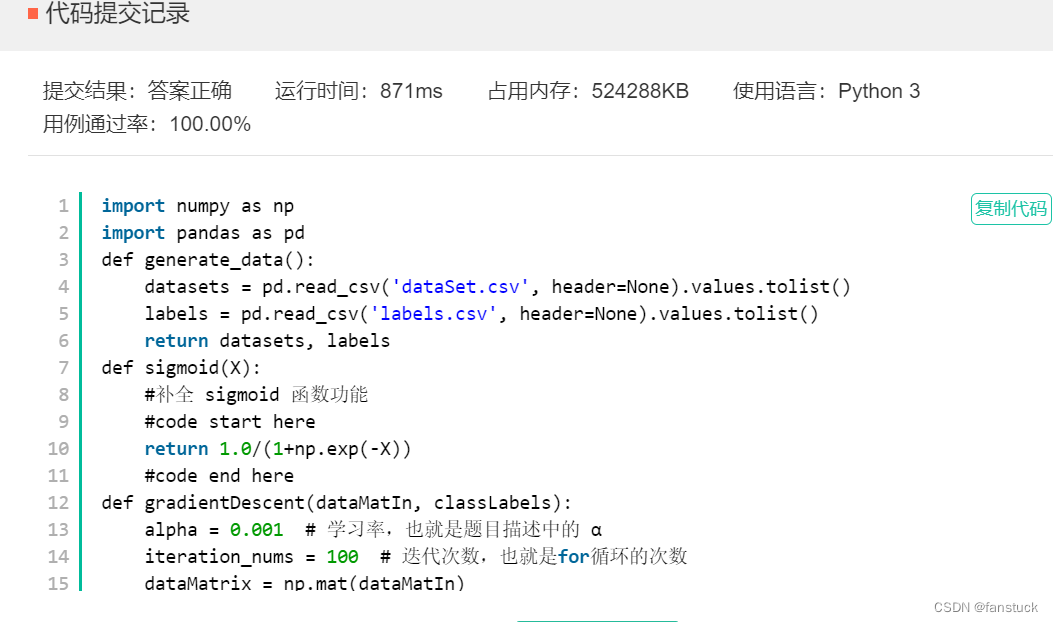刷题传送门：牛客刷题